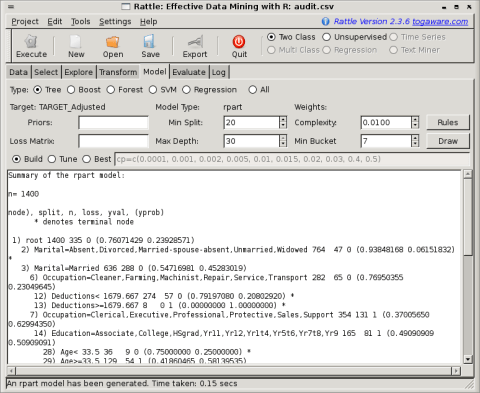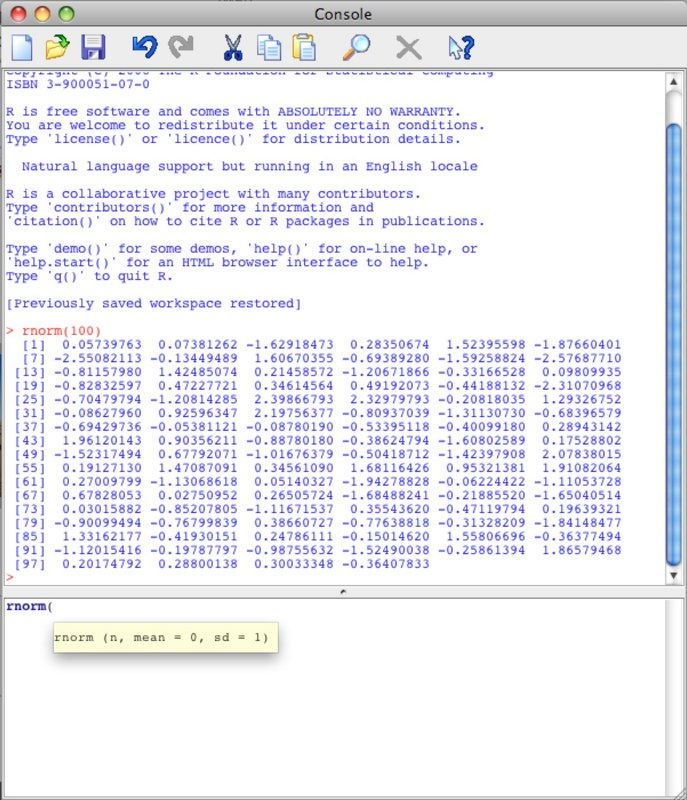# Graphical User Interfaces for R

## 1) R Commander

http://socserv.mcmaster.ca/jfox/Misc/Rcmdr/

Official Screenshot-Install-

Start R

>library(Rcmdr)

## R Commander Menu Tree [current as of version 1.6-0]

```File - Change working directory
|- Open script file
|- Save script
|- Save script as
|- Save output
|- Save output as
|- Save R workspace
|- Save R workspace as
|- Exit - from Commander
|- from Commander and R
Edit - Cut
|- Copy
|- Paste
|- Delete
|- Find
|- Select all
|- Undo
|- Redo
|- Clear Window
Data - New data set
|- Merge data sets
|- Import data - from text file, clipboard, or URL
|             |- from SPSS data set
|             |- from Minitab data set
|             |- from STATA data set
|             |- from Excel, Access, or dBase data set
|- Data in packages - List data sets in packages
|                  |- Read data set from attached package
|- Active data set - Select active data set
|                 |- Refresh active data set
|                 |- Help on active data set (if available)
|                 |- Variables in active data set
|                 |- Set case names
|                 |- Subset active data set
|                 |- Aggregate variables in active data set
|                 |- Remove row(s) from active data set
|                 |- Stack variables in active data set
|                 |- Remove cases with missing data
|                 |- Save active data set
|                 |- Export active data set
|- Manage variables in active data set - Recode variable
|- Compute new variable
|- Add observation numbers to data set
|- Standardize variables
|- Convert numeric variables to factors
|- Bin numeric variable
|- Reorder factor levels
|- Define contrasts for a factor
|- Rename variables
|- Delete variables from data set
Statistics - Summaries - Active data set
|           |- Numerical summaries
|           |- Frequency distributions
|           |- Count missing observations
|           |- Table of statistics
|           |- Correlation matrix
|           |- Correlation test
|           |- Shapiro-Wilk test of normality
|- Contingency Tables - Two-way table
|                    |- Multi-way table
|                    |- Enter and analyze two-way table
|- Means - Single-sample t-test
|       |- Independent-samples t-test
|       |- Paired t-test
|       |- One-way ANOVA
|       |- Multi-way ANOVA
|- Proportions - Single-sample proportion test
|             |- Two-sample proportions test
|- Variances - Two-variances F-test
|           |- Bartlett's test
|           |- Levene's test
|- Nonparametric tests - Two-sample Wilcoxon test
|                     |- Paired-samples Wilcoxon test
|                     |- Kruskal-Wallis test
|                     |- Friedman rank-sum test
|- Dimensional analysis - Scale reliability
|                      |- Principal-components analysis
|                      |- Factor analysis
|                      |- Cluster analysis - k-means cluster analysis
|                                         |- Hierarchical cluster analysis
|                                         |- Summarize hierarchical clustering
|                                         |- Add hierarchical clustering to data set
|- Fit models - Linear regression
|- Linear model
|- Generalized linear model
|- Multinomial logit model
|- Ordinal regression model
Graphs - Color palette
|- Index plot
|- Histogram
|- Stem-and-leaf display
|- Boxplot
|- Quantile-comparison plot
|- Scatterplot
|- Scatterplot matrix
|- Line graph
|- XY conditioning plot
|- Plot of means
|- Strip chart
|- Bar graph
|- Pie chart
|- 3D graph - 3D scatterplot
|          |- Identify observations with mouse
|          |- Save graph to file
|- Save graph to file - as bitmap
|- as PDF/Postscript/EPS
|- 3D RGL graph
Models - Select active model
|- Summarize model
|- Add observation statistics to data
|- Confidence intervals
|- Akaike Information Criterion (AIC)
|- Bayesian Information Criterion (BIC)
|- Stepwise model selection
|- Subset model selection
|- Hypothesis tests - ANOVA table
|                  |- Compare two models
|                  |- Linear hypothesis
|- Numerical diagnostics - Variance-inflation factors
|                       |- Breusch-Pagan test for heteroscedasticity
|                       |- Durbin-Watson test for autocorrelation
|                       |- RESET test for nonlinearity
|                       |- Bonferroni outlier test
|- Graphs - Basic diagnostic plots
|- Residual quantile-comparison plot
|- Component+residual plots
|- Influence plot
|- Effect plots
Distributions - Continuous distributions - Normal distribution - Normal quantiles
|            |                     |- Normal probabilities
|            |                     |- Plot normal distribution
|            |                     |- Sample from normal distribution
|            |- t distribution - t quantiles
|            |                |- t probabilities
|            |                |- Plot t distribution
|            |                |- Sample from t distribution
|            |- Chi-squared distribution - Chi-squared quantiles
|            |                          |- Chi-squared probabilities
|            |                          |- Plot chi-squared distribution
|            |                          |- Sample from chi-squared distribution
|            |- F distribution - F quantiles
|            |                |- F probabilities
|            |                |- Plot F distribution
|            |                |- Sample from F distribution
|            |- Exponential distribution - Exponential quantiles
|            |                          |- Exponential probabilities
|            |                          |- Plot exponential distribution
|            |                          |- Sample from exponential distribution
|            |- Uniform distribution - Uniform quantiles
|            |                      |- Uniform probabilities
|            |                      |- Plot uniform distribution
|            |                      |- Sample from uniform distribution
|            |- Beta distribution - Beta quantiles
|            |                   |- Beta probabilities
|            |                   |- Plot beta distribution
|            |                   |- Sample from beta distribution
|            |- Cauchy distribution - Cauchy quantiles
|            |                     |- Cauchy probabilities
|            |                     |- Plot Cauchy distribution
|            |                     |- Sample from Cauchy distribution
|            |- Logistic distribution - Logistic quantiles
|            |                       |- Logistic probabilities
|            |                       |- Plot logistic distribution
|            |                       |- Sample from logistic distribution
|            |- Lognormal distribution - Lognormal quantiles
|            |                        |- Lognormal probabilities
|            |                        |- Plot lognormal distribution
|            |                        |- Sample from lognormal distribution
|            |- Gamma distribution - Gamma quantiles
|            |                    |- Gamma probabilities
|            |                    |- Plot gamma distribution
|            |                    |- Sample from gamma distribution
|            |- Weibull distribution - Weibull quantiles
|            |                      |- Weibull probabilities
|            |                      |- Sample from Weibull distribution
|            |- Gumbel distribution - Gumbel quantiles
|                                  |- Gumbel probabilities
|                                  |- Plot Gumbel distribution
|                                  |- Sample from Gumbel distribution
|- Discrete distributions - Binomial distribution - Binomial quantiles
|                       |- Binomial tail probabilities
|                       |- Binomial probabilities
|                       |- Plot binomial distribution
|                       |- Sample from binomial distribution
|- Poisson distribution - Poisson quantiles
|                     |- Poisson tail probabilities
|                     |- Poisson probabilities
|                     |- Plot Poisson distribution
|                     |- Sample from Poisson distribution
|- Geometric distribution - Geometric quantiles
|                       |- Geometric tail probabilities
|                       |- Geometric probabilities
|                       |- Plot geometric distribution
|                       |- Sample from geometric distribution
|- Hypergeometric distribution - Hypergeometric quantiles
|                     |- Hypergeometric tail probabilities
|                     |- Hypergeometric probabilities
|                     |- Plot hypergeometric distribution
|                     |- Sample from hypergeometric distribution
|- Negative binomial distribution - Negative binomial quantiles
|- Negative binomial tail probabilities
|- Negative binomial probabilities
|- Plot negative binomial distribution
|- Sample from negative binomial distribution
|- Options
Help - Commander help
|- Introduction to the R Commander
|- Help on active data set (if available)
|- Start R help system```

## R Commander Plugins-

 Rcmdr R Commander RcmdrPlugin.DoE R Commander Plugin for (industrial) Design of Experiments RcmdrPlugin.EHESsampling Tools for sampling in European Health Examination Surveys (EHES) RcmdrPlugin.Export Graphically export output to LaTeX or HTML RcmdrPlugin.FactoMineR Graphical User Interface for FactoMineR RcmdrPlugin.HH Rcmdr support for the HH package RcmdrPlugin.IPSUR An IPSUR Plugin for the R Commander RcmdrPlugin.MAc Meta-Analysis with Correlations (MAc) Rcmdr Plug-in RcmdrPlugin.MAd Meta-Analysis with Mean Differences (MAd) Rcmdr Plug-in RcmdrPlugin.PT Some discrete exponential dispersion models: Poisson-Tweedie RcmdrPlugin.SLC SLC Rcmdr Plug-in RcmdrPlugin.SensoMineR Graphical User Interface for SensoMineR RcmdrPlugin.SurvivalT Rcmdr Survival Plug-In RcmdrPlugin.TeachingDemos Rcmdr Teaching Demos Plug-In RcmdrPlugin.TextMining Rcommander plugin for “tm” package RcmdrPlugin.doex Rcmdr plugin for Stat 4309 course RcmdrPlugin.epack Rcmdr plugin for time series RcmdrPlugin.orloca orloca Rcmdr Plug-in RcmdrPlugin.qcc Rcmdr qcc Plug-In RcmdrPlugin.qual Rcmdr plugin for quality control course RcmdrPlugin.sos Efficiently search R Help pages RcmdrPlugin.steepness Steepness Rcmdr Plug-in RcmdrPlugin.survival R Commander Plug-in for the survival Package

2) Rattle

Official Screenshot-## 3) Deducer

File Data Analysis Packages & Data Plots
Open Data Edit Factor Frequencies Data Viewer Plot Builder
Save Data Recode Variables Descriptives Import Template
Transform Contingency Tables Open Plot
Reset Row Names One Sample Test Templates
Sort Two Sample Test Interactive
Transpose K-Sample Test
Merge Data Correlation
Subset Linear Model
Logistic Model
Generalized Linear Model

Official Screenshot-## Extension Packages

DeducerExtras
An add-on package containing a variety of additional analysis dialogs. These include: Distribution quantiles, single/multiple sample proportion tests, paired t-test, Wilcoxon signed rank test, Levene’s test, Bartlett’s test, k-means clustering, Hierarchical clustering, factor analysis, and multi-dimensional scaling
DeducerPlugInScaling
Reliability and factor analysis
gMCP
(Experimental) A graphical approach to sequentially rejective multiple test procedures
RGG
A GUI Generator

## 4) RKWard

http://rkward.sourceforge.net/

Install-

Official Screenshots-

http://sourceforge.net/apps/mediawiki/rkward/index.php?title=Screenshots## 5) Red-R

http://red-r.org/

Install-

Official Screenshot-## 6) GrapheR

Home-

http://cran.r-project.org/web/packages/GrapheR/index.html

Install-

http://cran.r-project.org/web/packages/GrapheR/GrapheR.pdf

(UN) Official Screenshot-## 7) Komodo Sciviews-K

http://sciviews.org/SciViews-K/

Official Screenshot-## 8  ) JGR

http://www.rforge.net/JGR/

Install-

https://www.rforge.net/JGR/files/

Official Screenshot-## 9) PMG

http://wiener.math.csi.cuny.edu/pmg/pmg/

(UN)Official Screenshot10) R Analytic Flow

http://www.ef-prime.com/products/ranalyticflow_en/index.html

Install-

Official Screenshot## 3 thoughts on “Graphical User Interfaces for R”

1.D.V.Deshpande

Fantastic

1.Ajay Ohri Post author

R Studio is an IDE not a proper GUI as of now